# Trigonometric Ratios

Trigonometric Ratios

The word "trigonometry" is a 16th century's Latin derivative.

The word "Trigonometry" originated from the words, "Trigonon" which means "triangle" and "Metron" which means "to measure."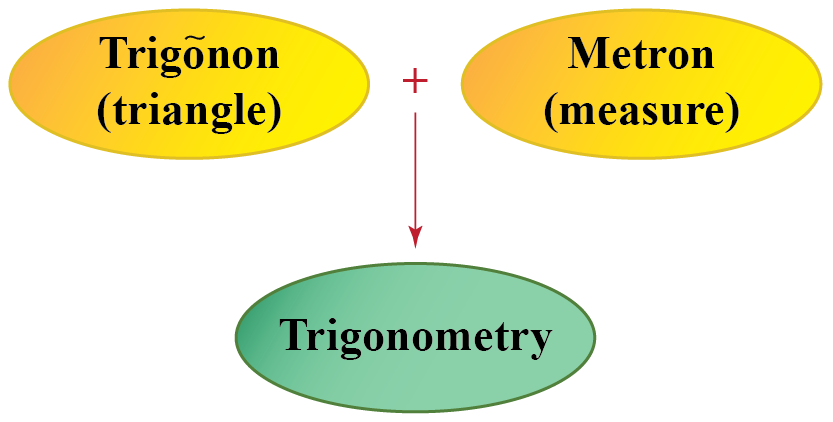It is a branch of mathematics that deals with the relation between the angles and sides of a triangle.

In fact, trigonometry is one of the most ancient subjects which is studied by scholars all over the world.

In this mini-lesson, we will learn about trigonometric identities, trigonometric ratio formulas, reciprocal relations for Trigonometric ratios, and various trigonometric functions like Sine, Cosine, and Tangent.

## Lesson Plan

 1 What Do You Mean by Trigonometric Ratios? 2 Important Notes on Trigonometric Ratios 3 Solved Examples on Trigonometric Ratios 4 Thinking out of the Box! 5 Interactive Questions on Trigonometric Ratios

## What Do You Mean by Trigonometric Ratios?

### Trigonometric Ratios Formulas

Look at the right-angled triangle shown below.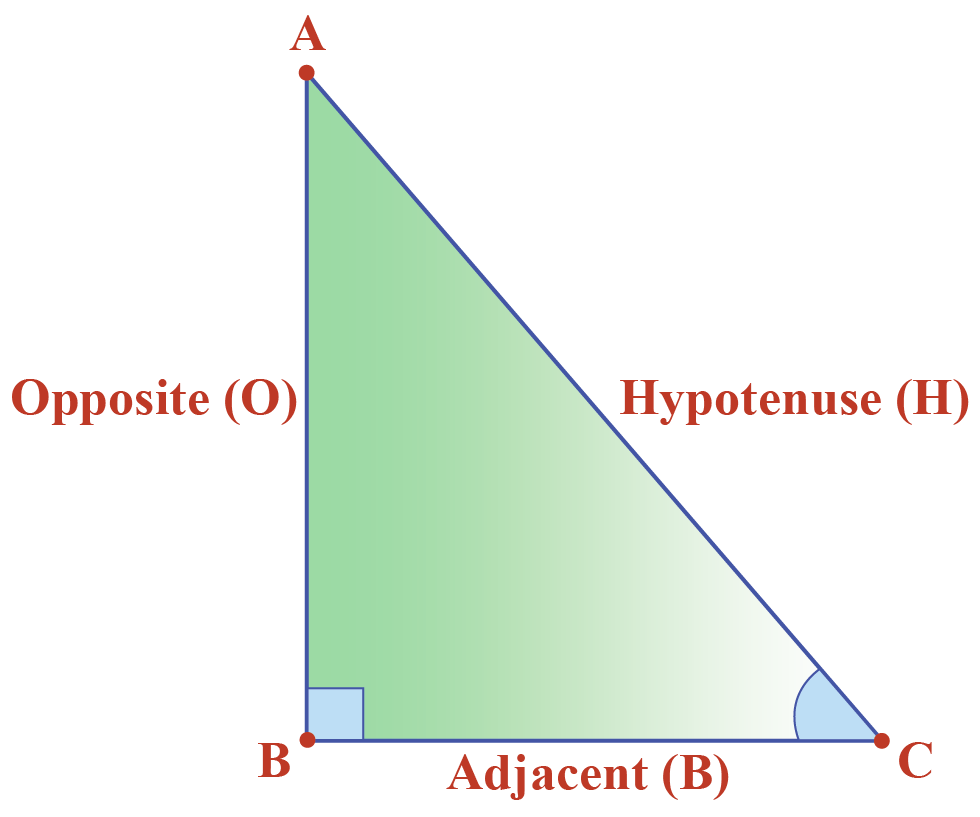How many different ratios of sides, $$P$$, $$B$$, and $$H$$ can you make?

In a ratio, we can take two sides at a time from a total of three sides of a right-angled triangle.

There are six possible ratios that can be defined for $$\theta$$.

$$\sin{\theta}=\dfrac{P}{H},\cos{\theta}=\dfrac{B}{H}, \tan{\theta}=\dfrac{P}{B},$$

$$\csc{\theta}=\dfrac{H}{P}, \sec{\theta}=\dfrac{B}{P}$$, and $$\cot{\theta}=\dfrac{B}{P}$$.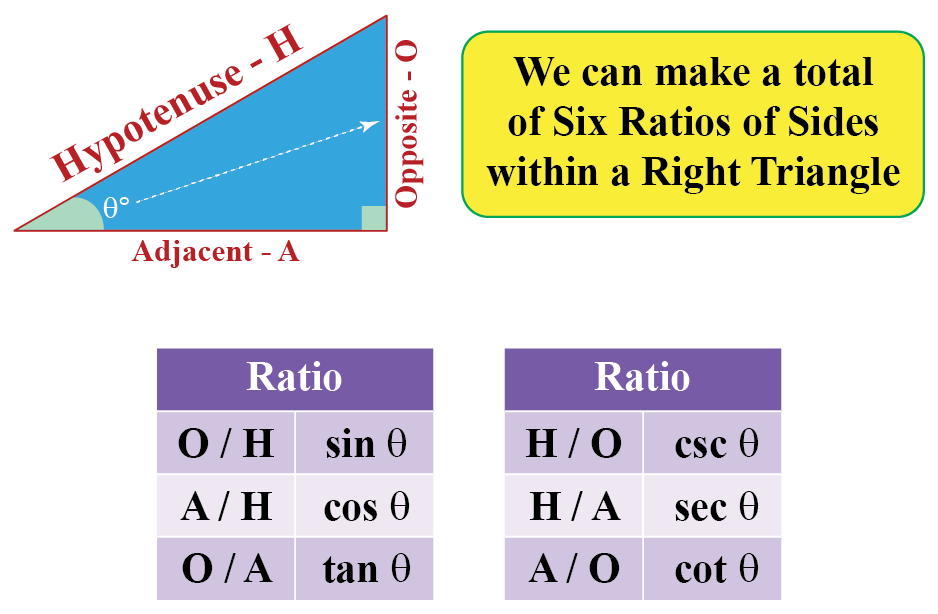### Reciprocal Relations For Trigonometric Ratios

Observe that

• $$\sin{\theta}$$ is the reciprocal of $$\csc{\theta}$$
•  $$\cos{\theta}$$ is the reciprocal of $$\csc{\theta}$$
• $$\tan{\theta}$$ is the reciprocal of $$\cot{\theta}$$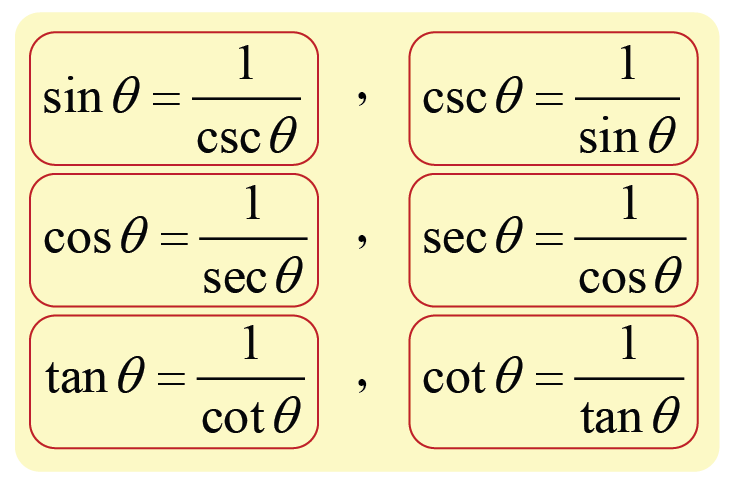## How to Find Trigonometric Ratios?

Let's do one example to find the trigonometric ratios.

Here, $$ABC$$ is a right-angled triangle with $$\tan{A}=\dfrac{5}{12}$$.

We will find all other trigonometric ratios of $$\angle A$$.

Given that, $$\tan{A}=\dfrac{BC}{AB}=\dfrac{5}{12}$$

Hence, we can write $$BC=5k$$ and $$AB=12k$$, where $$k$$ is a positive integer.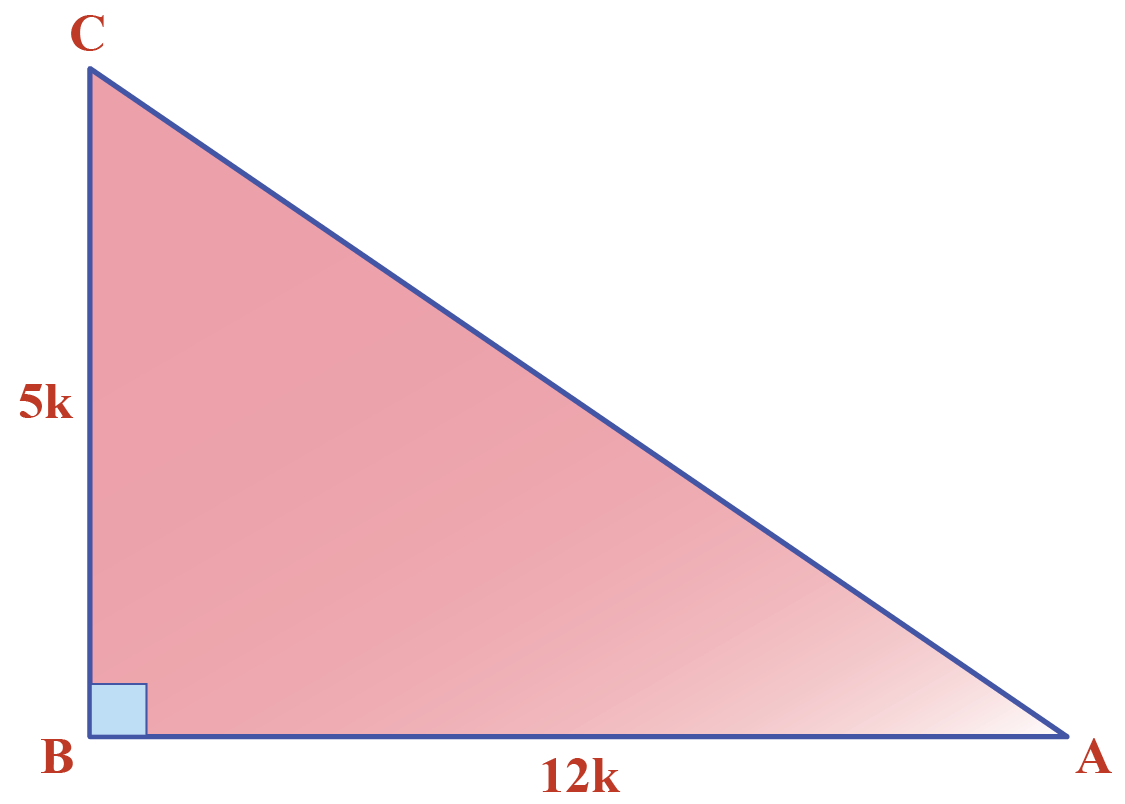Let's use Pythagoras theorem to find the other trigonometric ratios.

\begin{align}(AC)^{2}&=(AB)^{2}+(BC)^{2}\\&=144k^{2}+25k^{2}\\&=169k^{2}\end{align}

Thus, $$AC=13k$$.

So, other trigonometric ratio are given below.

$\sin{A}=\dfrac{BC}{AC}=\dfrac{5k}{13k}=\dfrac{5}{13}$

$\cos{A}=\dfrac{AB}{AC}=\dfrac{12k}{13k}=\dfrac{12}{13}$

$\cot{A}=\dfrac{1}{\tan{A}}=\dfrac{12}{5}$

$\csc{A}=\dfrac{1}{\sin{A}}=\dfrac{13}{5}$

$\sec{A}=\dfrac{1}{\cos{A}}=\dfrac{13}{12}$

Check out this simulation to experiment with trigonometric ratios.

Drag the points to change the side lengths of the right-angled triangle.

## Formulas for Trigonometric Ratios

Let's define trigonometric ratios for the right-angled triangled shown below.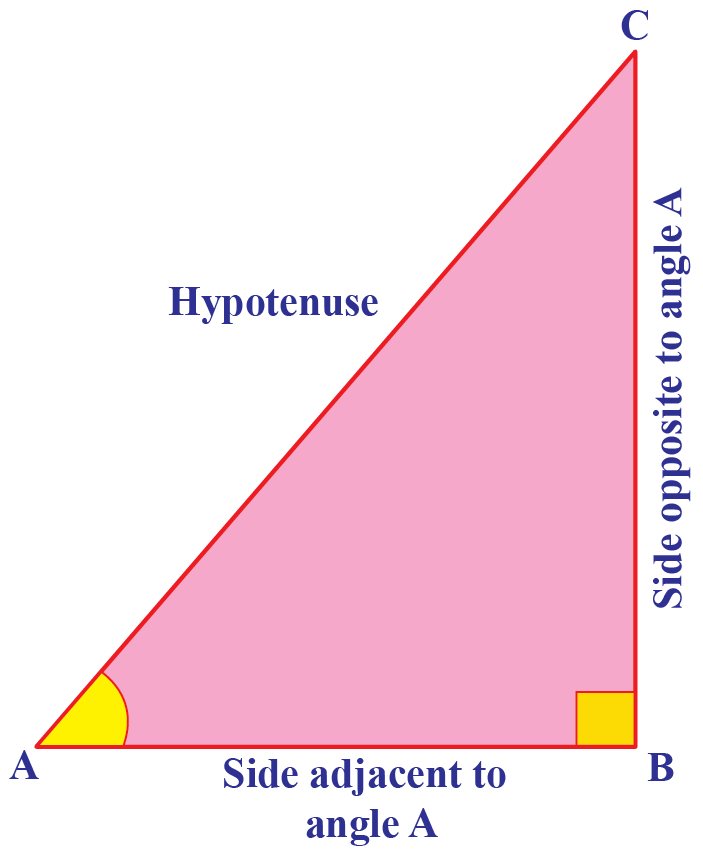### Trigonometric Ratio: Formulas

We will now define some ratios involving the sides of the triangle $$ABC$$.

 $$\sin{A}=\dfrac{\text{Side Opposite to } \angle A}{\text{Hypotenuse}}=\dfrac{BC}{AC}$$
 $$\cos{A}=\dfrac{\text{Side Adjacent to } \angle A}{\text{Hypotenuse}}=\dfrac{AB}{AC}$$
 $$\tan{A}=\dfrac{\text{Side Opposite to } \angle A}{\text{Side Adjacent to }\angle A}=\dfrac{BC}{AB}$$
 $$\csc{A}=\dfrac{1}{\sin A}=\dfrac{AC}{BC}$$
 $$\sec{A}=\dfrac{1}{\cos A}=\dfrac{AC}{AB}$$
 $$\cot{A}=\dfrac{1}{\tan A}=\dfrac{AB}{BC}$$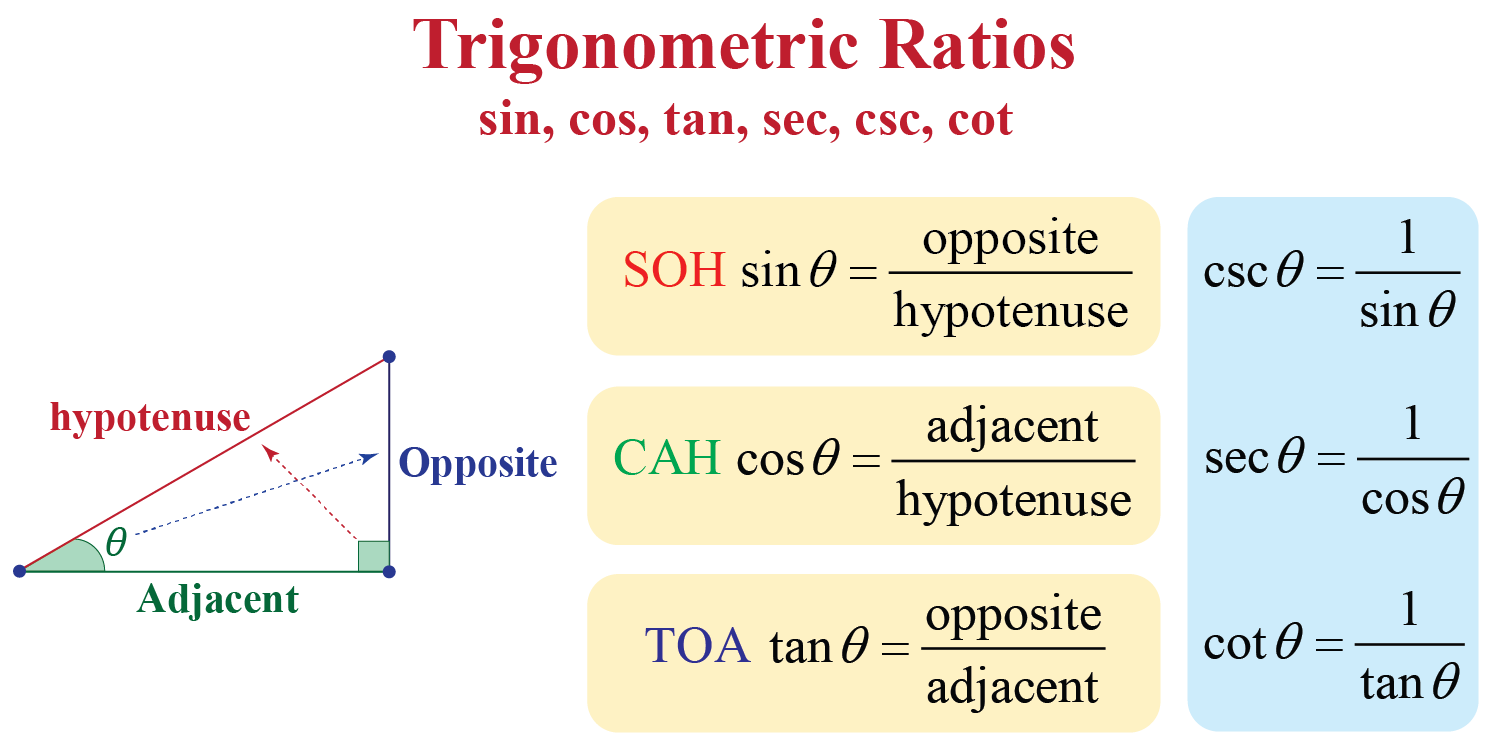## Trigonometric Ratios Table

You are already familiar with construction of angles like $$30^{\circ}$$, $$45^{\circ}$$, $$60^{\circ}$$, and $$90^{\circ}$$.

We will now see the values in the trigonometric chart for these angles.

$$\angle A$$ $$0^{\circ}$$ $$30^{\circ}$$ $$45^{\circ}$$ $$60^{\circ}$$ $$90^{\circ}$$
$$\sin {A}$$ 0 $$\dfrac{1}{2}$$ $$\dfrac{1}{\sqrt{2}}$$ $$\dfrac{\sqrt{3}}{2}$$ 1
$$\cos {A}$$ 1 $$\dfrac{\sqrt{3}}{2}$$ $$\dfrac{1}{\sqrt{2}}$$ $$\dfrac{1}{2}$$ 0
$$\tan {A}$$ 0 $$\dfrac{1}{\sqrt{3}}$$ 1 $$\sqrt{3}$$ Not defined
$$\csc {A}$$ Not defined 2 $$\sqrt{2}$$ $$\dfrac{2}{\sqrt{3}}$$ 1
$$\sec {A}$$ 1 $$\dfrac{2}{\sqrt{3}}$$ $$\sqrt{2}$$ 2 Not defined
$$\cot {A}$$ Not defined $$\sqrt{3}$$ 1 $$\dfrac{1}{\sqrt{3}}$$ 0

## Trigonometric Identities

Here are the basic trigonometric identities which are true for all angles involved in a right-angled triangle.

 $$\cos^{2}{A}+\sin^{2}{A}=1$$
 $$1+\tan^{2}{A}=\sec^{2}{A}$$
 $$1+\cot^{2}{A}=\csc^{2}{A}$$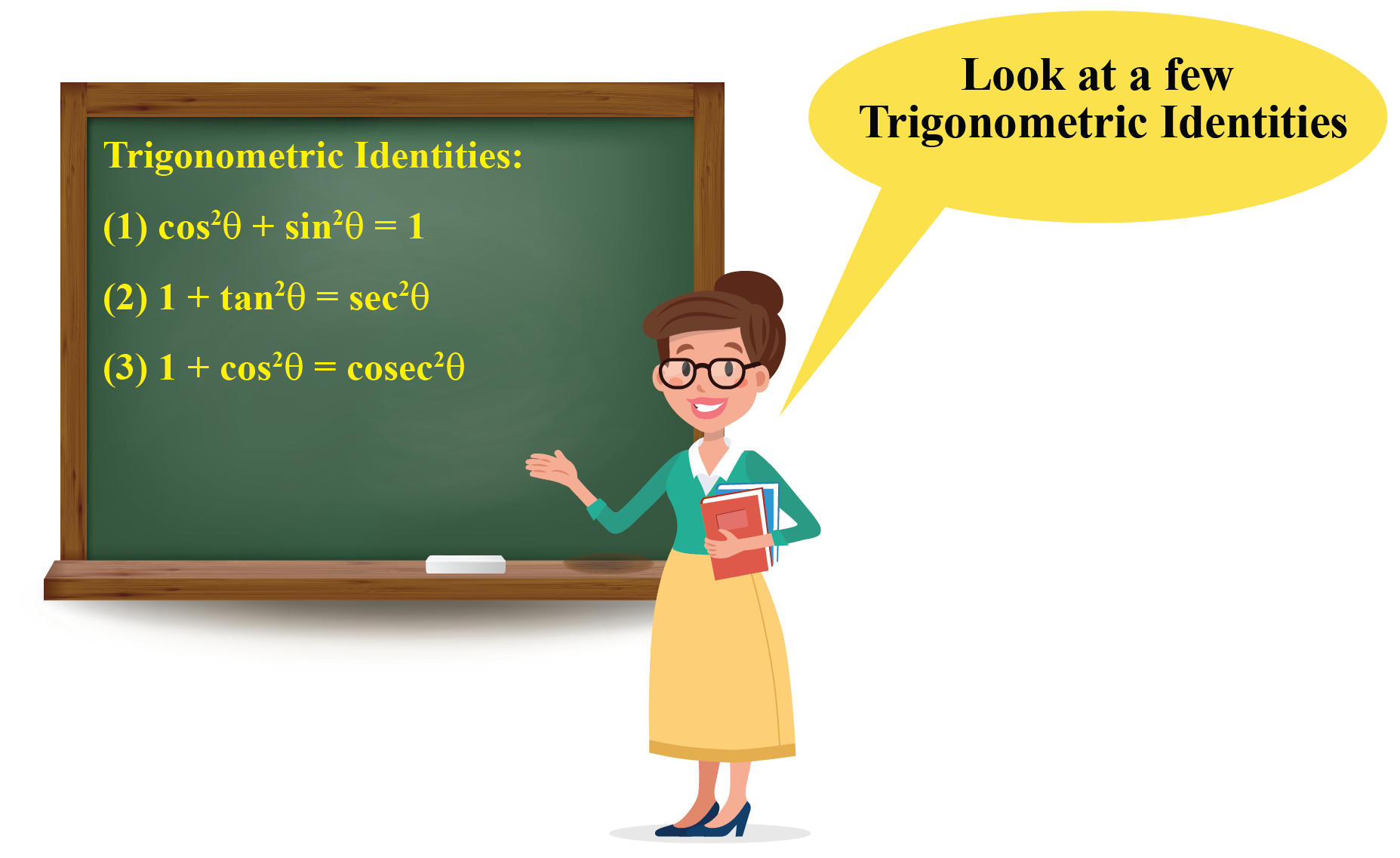## Solved Examples

 Example 1

The building is at a distance of 150 feet from point A.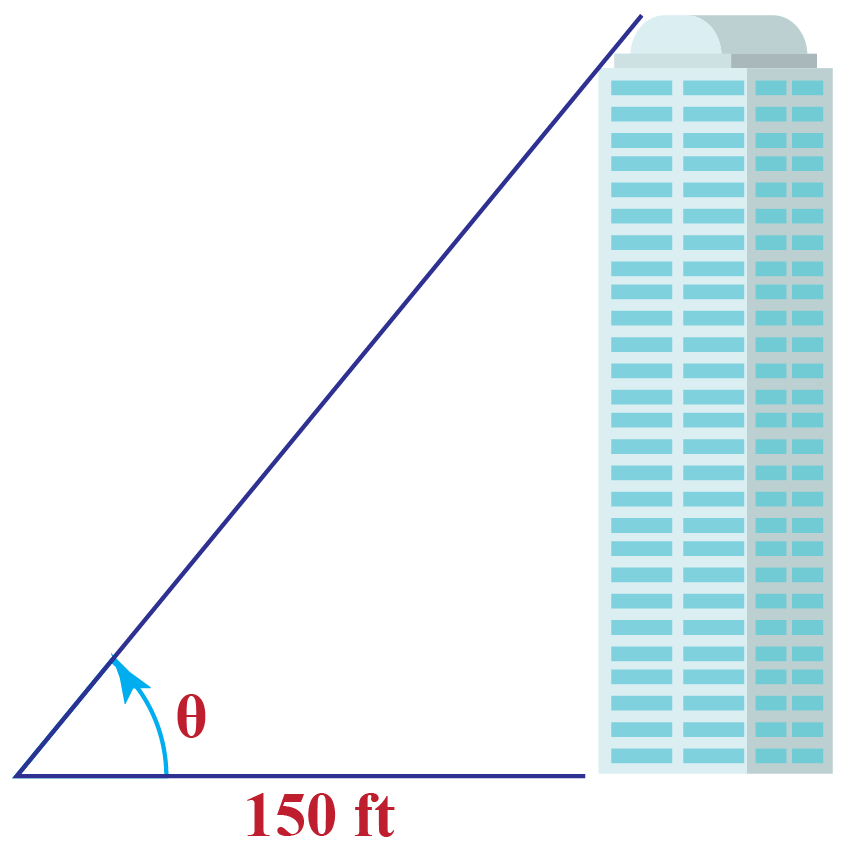Can you calculate the height of this building if $$\tan{\theta}=\dfrac{4}{3}$$?

### Solution

Clearly, the triangle formed here is a right-angle triangle.

Now apply the trigonometric ratio of $$\tan{\theta}$$ to calculate the height of the building.

\begin{align}\tan{\theta}&=\left(\dfrac{\text{Opposite}}{\text{Adjacent}}\right)\\\dfrac{4}{3}&=\left(\dfrac{\text{Height}}{150\;\text{ft}}\right)\\\text{Height}&=\left(\dfrac{4\times 150}{3}\right)\;\text{ft}\\&=200\;\text{ft}\end{align}

 $$\therefore$$ The height of the building is $$200\;\text{ft}$$.
 Example 2

Look at the triangle below.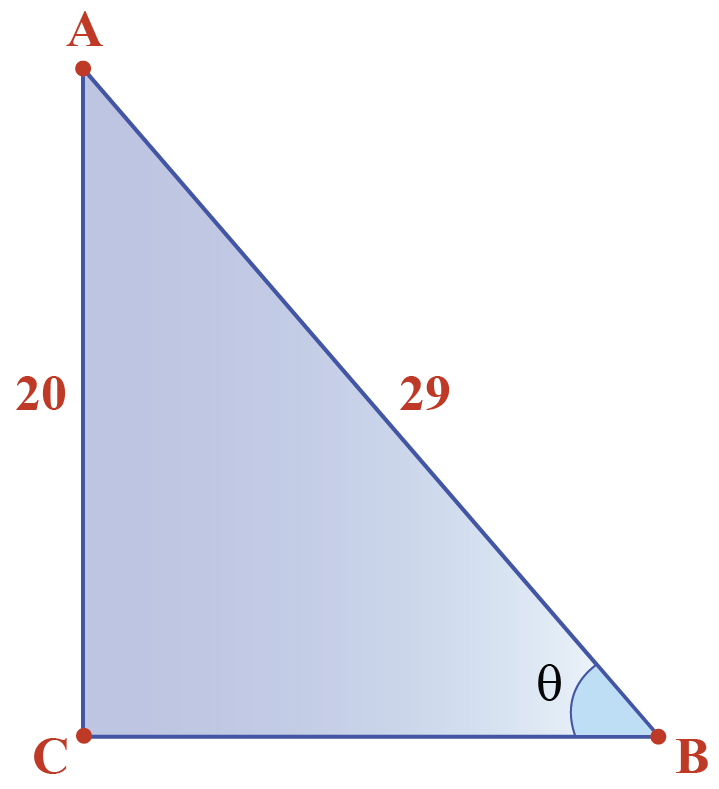The triangle is right-angled at $$C$$ with $$AB=29\;\text{units}$$ and $$AC=20\;\text{units}$$.

Can you verify the trigonometric identity $$\cos^{2}{A}+\sin^{2}{A}=1$$ using these values?

Solution

We will find $$BC$$ using Pythagoras theorem.

\begin{align}BC&=\sqrt{(AB)^{2}-(AC)^{2}}\\&=\sqrt{29^{2}-20^{2}}\\&=\sqrt{841-400}\\&=\sqrt{441}\\&=21\end{align}

Now let's determine the values of $$\sin{\theta}$$ and $$\cos{\theta}$$.

$\sin{\theta}=\dfrac{AC}{AB}=\dfrac{20}{29}$

$\cos{\theta}=\dfrac{BC}{AB}=\dfrac{21}{29}$

Now let's verify the identity.

\begin{align}\cos^{2}{\theta}+\sin^{2}{\theta}&=\left(\dfrac{21}{29}\right)^{2}+\left(\dfrac{20}{29}\right)^{2}\\&=\dfrac{400+441}{841}\\&=1\end{align}

 Thus, the identity is verified.
 Example 3

In a $$\Delta ABC$$, $$\angle A=60^{\circ}$$ and  $$\angle B=90^{\circ}$$. Also $$AC=4 \text{ units}$$. It is known that $$\sec{60^{\circ}}=2$$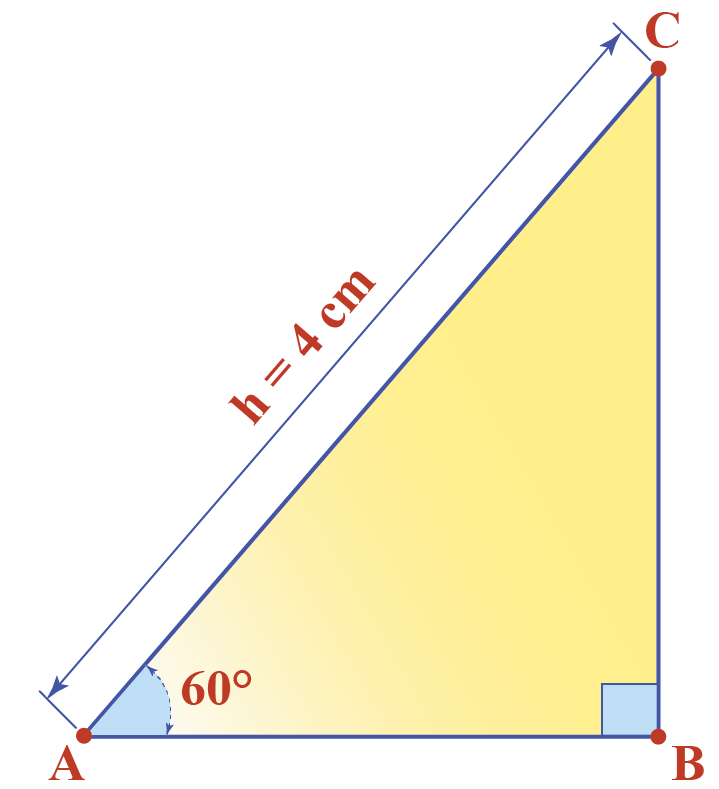Find the length of AB and BC.

Solution

Use the trigonometric ratio formula $$\sec{\theta}=\dfrac{\text{Hypotenuse}}{\text{Adjacent}}$$ and solve it for AB.

\begin{aligned}\sec{\theta}&=\dfrac{\text{Hypotenuse}}{\text{Adjacent}}\\\sec{60^{\circ}}&=\dfrac{AC}{AB}\\2&=\dfrac{4}{AB}\\AB&=2\text{ units}\end{aligned}

Now use the Pythagorean theorem to calculate the side BC.

\begin{aligned}BC&=\sqrt{(AC)^2-(AB)^2}\\&=\sqrt{(4)^2-(2)^2}\\&=\sqrt{16-4}\\&=\sqrt{12}\\&=2\sqrt{3}\text{ units}\end{aligned}

 So, $$AB = 2 \text{ units}$$ and BC = $$2\sqrt{3}$$ unitsThink Tank

 1. Two right triangles are given below.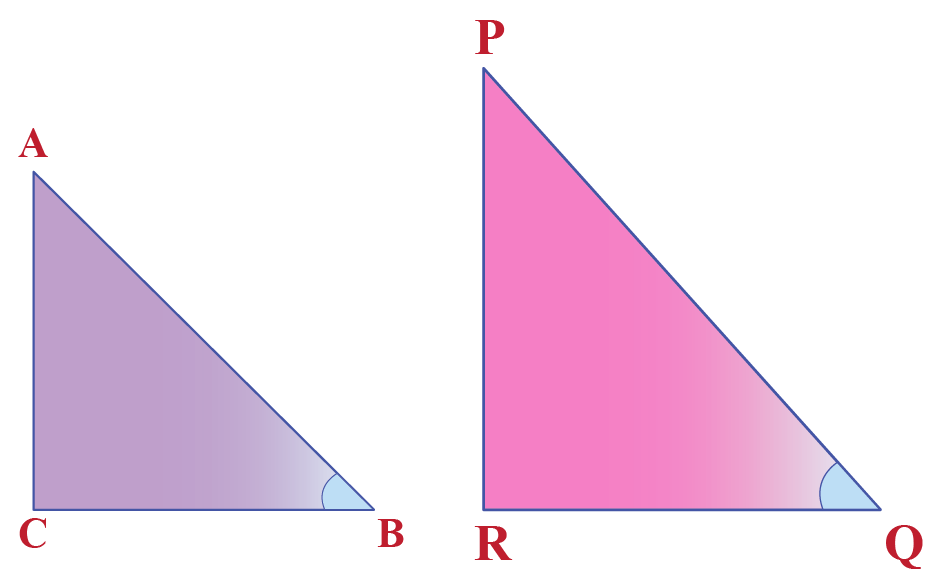If $$\angle B$$ and $$\angle Q$$ are acute angles that satisfy $$\sin B=\sin Q$$, can you prove that $$\angle B=\angle Q$$?

## Interactive Questions

Here are a few activities for you to practice.

Select/type your answer and click the "Check Answer" button to see the result.

## Let's Summarize

The mini-lesson targeted the fascinating concept of Trigonometric Ratios. The math journey around Trigonometric Ratios starts with what a student already knows, and goes on to creatively crafting a fresh concept in the young minds. Done in a way that not only it is relatable and easy to grasp, but also will stay with them forever. Here lies the magic with Cuemath.

## About Cuemath

At Cuemath, our team of math experts is dedicated to making learning fun for our favorite readers, the students!

Through an interactive and engaging learning-teaching-learning approach, the teachers explore all angles of a topic.

Be it worksheets, online classes, doubt sessions, or any other form of relation, it’s the logical thinking and smart learning approach that we, at Cuemath, believe in.

## 1. What are the applications of trigonometric ratios?

In astronomy, it is used to determine the distances from the Earth to the planets and stars.

In geography and navigation, it is used to construct maps.

It is also used to find the position of an island in relation to the longitudes and latitudes.

Even today, some of the technologically advanced methods which are used in engineering and physical sciences are based on the concepts of trigonometry.

## 2. What is adjacent over the opposite?

We know that $$\tan{\theta}=\dfrac{\text{Opposite}}{\text{Adjacent}}$$.

Now, the reciprocal of $$\tan{\theta}$$ is equal to $$\cot{\theta}$$.

Therefore,  $$\dfrac{\text{Adjacent}}{\text{Opposite}}=\cot{\theta}$$.

## 3. What is the value of sin 15?

The value of $$\sin{15^{\circ}}$$ is equal to $$\dfrac{\sqrt{3}-1}{2\sqrt{2}}=0.2588...$$

## 4. What is the formula for tan?

The formula of tangent of an angle is, $$\tan{\theta}=\dfrac{\text{Opposite}}{\text{Adjacent}}$$.

Download Trigonometry Worksheets
Trigonometry
Grade 10 | Answers Set 1
Trigonometry
Grade 9 | Questions Set 1
Trigonometry
Grade 10 | Questions Set 1
Trigonometry
Grade 9 | Answers Set 1
More Important Topics
Numbers
Algebra
Geometry
Measurement
Money
Data
Trigonometry
Calculus